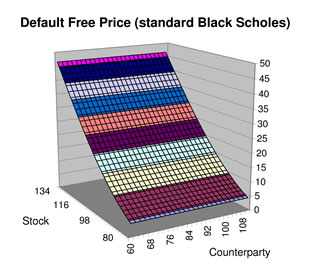# The Black-Scholes Option Pricing FormulaDerived by Myron Scholes, Robert Merton, and the late Fischer Black, the Black-Scholes Option Pricing Formula is a way to determine how much a call option is worth at any given time.

Certain economists liken the impact of its discovery, which earned Scholes and Merton the 1997 Nobel Prize in Economics, to that of the discovery of the structure of DNA.

Both gave birth to new fields of immense practical importance: genetic engineering on the one hand and, on the other, financial engineering.

The latter relies on risk-management strategies, such as the use of the Black-Scholes formula, to reduce our vulnerability to the financial insecurity generated by a rapidly changing global economy.

When a call option on a stock expires, its value is either zero (if the stock price is less than the exercise price) or the difference between the stock price and the exercise price of the option.

For example, say you buy a call option on XYZ stock with an exercise price of 100. If at the option’s expiration date the price of XYZ stock is less than 100, the option is worthless.

If, however, the stock price is greater than 100 — say 120, then the call option is worth 20. The higher the stock price, the more the option is worth. The difference between the stock price and the exercise price is the “payoff” to the call option.

The Black-Scholes Formula was derived by observing that an investor can precisely replicate the payoff to a call option by buying the underlying stock and financing part of the stock purchase by borrowing.

To understand this, consider our example of XYZ stock. Suppose that instead of owning the call option, you purchased a share of XYZ stock itself and borrowed the 100 exercise price.

At the option’s expiration date, you sell the stock for 120, you pay back the 100 loan, and you are left with the 20 difference less the interest on the loan.

Note that at any price above the 100 exercise price, this equivalence exists between the payoff from the call option and the payoff from the so-called “replicating portfolio.”

But what about before the call option expires? Believe it or not, you can still match its future payoff by creating a replicating portfolio.

However, to do so you must buy a fraction of a share of the stock and borrow a fraction of the exercise price.

How do you know what these fractions are?

That is what the Black-Scholes Formula tells you!

It states that the price of the call option is equal to a fraction of the stock’s current price, minus a fraction of the exercise price.

The fractions depend on five factors, four of which are directly observable. They are:

1. The Price of the Stock

2. The Exercise Price of the Option

3. The Risk-Free Interest Rate (the annualized, continuously compounded rate on a safe asset with the same maturity as the option) and

4. The Time to Maturity of the Option

The only unobservable is the volatility of the underlying stock price.

If the current stock price is way above the exercise price, these fractions are close to 1, and therefore the call option is approximately the difference between the stock’s current price and the present discounted value of the exercise price.

If, on the other hand, the current stock price is way below the exercise price, these fractions are close to zero, making the value of the call option very low.

(Text in part by www.pbs.org)